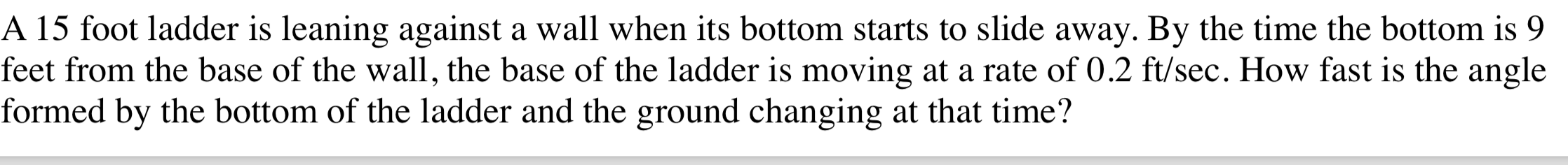# A 15 foot ladder is leaning against a wall when its bottom starts to slide away. By the time the bottom is 9feet from the base of the wall, the base of the ladder is moving at a rate of 0.2 ft/sec. How fast is the angleformed by the bottom of the ladder and the ground changing at that time?

Question
889 viewshelp_outlineImage TranscriptioncloseA 15 foot ladder is leaning against a wall when its bottom starts to slide away. By the time the bottom is 9 feet from the base of the wall, the base of the ladder is moving at a rate of 0.2 ft/sec. How fast is the angle formed by the bottom of the ladder and the ground changing at that time? fullscreen
check_circle

Step 1

Please refer to the diagram on the white board.

l= Length of the ladder = 15 ft

x = lcosϴ

At the moment, we are at x = 9 ft.

Hence, cosϴ = x / l = 9 / 15 = 3 / 5

Hence, sin2ϴ = 1 - cos2ϴ = 1 - (3 / 5)2 = 16 / 25

Hence, sinϴ = 4 / 5

x will increase at the rate of dx/dt

y will decrease at the rate of dy / dt

and ϴ, the angle between the bottom of the ladder and the ground will decrease by dϴ / dt

Step 2

Step 3

dϴ / dt = -(1 / lsinϴ).dx / dt

l = 15 ft; sinϴ = 4 / 5 and dx / dt = 0.2 ft / sec

lsinϴ = 15 x...

### Want to see the full answer?

See Solution

#### Want to see this answer and more?

Solutions are written by subject experts who are available 24/7. Questions are typically answered within 1 hour.*

See Solution
*Response times may vary by subject and question.
Tagged in

### Derivative